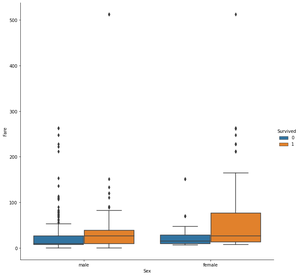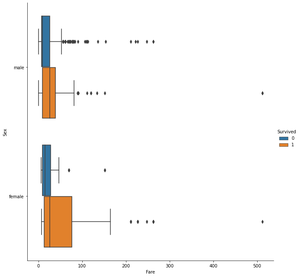# How To Make Grouped Boxplot with Seaborn Catplot?

• Difficulty Level : Expert
• Last Updated : 12 Nov, 2020

Prerequisite: seaborn

A grouped boxplot is a boxplot where categories are organized in groups and subgroups. Whenever we want to visualize data in the group and subgroup format the Seaborn Catplot() plays a major role. The following example visualizes the distribution of 7 groups (called A to G) and 2 subgroups (called low and high) in grouped boxplot format. To generate boxplot using Seaborn generally uses the boxplot() method but here we use a much newer method Catplot(). The Catplot() accesses several axes-level functions that show the relationship between a numerical and one or more categorical variables using one of several visual representations.

### Grouped Boxplot

In this article, we will learn how to generates Grouped Boxplot using Seaborn Catplot. Please follow the steps mentioned below –

• Import required packages.
• Load the dataset.
• Now use catplot() method which is available within the seaborn package. Let’s pass the x and y variable, here the variable on the x-axis is continuous and the variable on the y-axis is categorical also pass other parameters like data, hue, height, aspect, and kind=” box”.

Syntax:

catplot(x, y, hue, data, height ,kind)

Dataset used: titanic_train.csv

Example 1: Horizontal Boxplot

## Python3

 `import` `pandas as pd``import` `seaborn as sns`` ` ` ` `df ``=` `pd.read_csv(``"titanic_train.csv"``)``df.dropna()``sns.catplot(x``=``'Sex'``, y``=``'Fare'``, hue``=``'Survived'``, ``            ``data``=``df, height``=``9``, kind``=``"box"``)`

Output :Example 2: Vertical Boxplot

This example depicts how we can plot the same data horizontally. This can be achieved simply by swapping values provided to x and y.

## Python3

 `import` `pandas as pd``import` `seaborn as sns`` ` ` ` `df ``=` `pd.read_csv(``"titanic_train.csv"``)``df.dropna()``sns.catplot(y``=``'Sex'``, x``=``'Fare'``, hue``=``'Survived'``,``            ``data``=``df, height``=``9``, kind``=``"box"``)`

Output:My Personal Notes arrow_drop_up